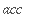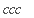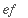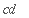Advice: The differences between cat and dot

There are two ways to join two strings together: with the cat function (e.g. cat(a,b) ) and with the dot operator (e.g. a.b ). In many situations these have identical results, but there are two differences:

The dot operator does not evaluate its first argument. The other arguments are evaluated. The cat function, on the other hand, evaluates all its arguments. In the example below, evaluating a results in c . The first a in a.a.a is not evaluated, but all the other a 's are.

> a:= b: b:= c:

> a.a.a;> cat(a,a,a);The result of the dot operator is evaluated, while the result of cat is not evaluated. In the example below, evaluating cd results in ef . Recalling the unevaluated result of cat with " evaluates it.

> cd:= ef:

> c.d;> cat(c,d);> ";